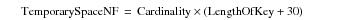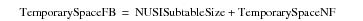# 15.00 - NUSI Space Considerations - Teradata Database

prodname
vrm_release
15.00
category
User Guide
featnum
B035-1094-015K

### NUSI Space Considerations

The PERM space required during the creation of a NUSI might temporarily be much greater than the space occupied by the finished index as described by the following table.

 IF you create this sort of table … THEN the following peak temporary PERM space usage factors apply … non-fallback or fallback with small AMP cluster size Estimate the temporary PERM space required when building a NUSI using the following worst case estimation equation.where: TemporarySpaceNF is the size of the temporary PERM space required without fallback. Cardinality is the number of rows in the base table. LengthOfKey is the combined byte length of the key. fallback Estimate the temporary PERM space required, assuming typical AMP cluster size, using the following equation.This is a very conservative space estimate. For typical AMP cluster sizes, peak usage exceeds the prediction made by the model.

AMP cluster size, which determines the relative size of the backup subtables, is a an important factor. For information about AMP clusters, see Introduction to Teradata. A typical AMP cluster size is 4 AMPs, but the valid range varies from 2 to 8 AMPs per cluster.

NUSIs do not use spool space and are built one subtable at a time.